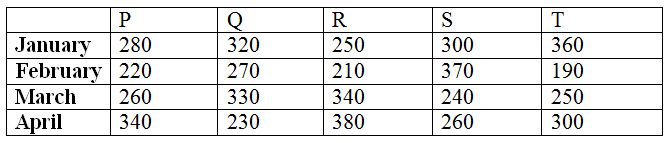# IBPS RRB Clerk/EPFO Assistant Prelims Quantitative Aptitude Questions 2019 (Day-02)

Dear Aspirants, Our IBPS Guide team is providing new series of Quantitative Aptitude Questions for IBPS RRB Clerk/EPFO Assistant Prelims 2019 so the aspirants can practice it on a daily basis. These questions are framed by our skilled experts after understanding your needs thoroughly. Aspirants can practice these new series questions daily to familiarize with the exact exam pattern and make your preparation effective.

##### IBPS EPFO Assistant Prelims Free Mock Test

[WpProQuiz 6554]

Directions (Q. 1 – 5): what approximate value should come in place of question mark (?) in the following questions?

1) (12.32)2 ÷ (3.15)2 × 80.11 ÷ 10.23 = ? – 411 ÷ 4.11

a) 230

b) 250

c) 220

d) 270

e) 200

2) (4.3 / 6.78) of 48.7 × 15 ÷ 5.12 + ? = 129.68

a) 66

b) 28

c) 82

d) 46

e) 74

3) √1291 × 24.91 ÷ 15.11 = ? – (8.11)2

a) 138

b) 124

c) 152

d) 166

e) 110

4) (2 / 7) of (21.2 / 13.8) of 188 = ? % of 299

a) 24

b) 31

c) 27

d) 32

e) 35

5) 13 4/5 + 8 3/10 – 11 1/5 + 16 7/10 = ? – 4 4/5

a) 33

b) 30

c) 36

d) 32

e) 35

Directions (Q. 6 – 10): Study the following information carefully and answer the given questions:

The following table shows the total number of birthday cakes sold by 5 different bakeries in 4 different months.6) Find the difference between the total number of cakes sold by bakery R and T together on January to that of total number of cakes sold by bakery P and S together on April?

a) 10

b) 25

c) 40

d) 55

e) None of these

7) Find the average number of cakes sold by bakery P on all the given months together?

a) 260

b) 290

c) 305

d) 275

e) None of these

8) Find the ratio between the total number of cakes sold by bakery Q on January and March together to that of total number of cakes sold by bakery S on March and April together?

a) 17 : 12

b) 13 : 10

c) 25 : 19

d) 34 : 27

e) None of these

9) Total number of cakes sold by bakery P, Q and R together on February is approximately what percentage of total number of cakes sold by bakery R, S and T together on March?

a) 96 %

b) 112 %

c) 84 %

d) 75 %

e) 60 %

10) If the total number of cakes sold by bakery P on May is 20 % more than the average number of cakes sold by bakery P on all the given months together, find the total number of cakes sold by bakery P on May?

a) 330

b) 350

c) 320

d) 360

e) None of these

Directions (1-5):

(12.32)2 ÷ (3.15)2 × 80.11 ÷ 10.23 = ? – 411 ÷ 4.11

(12)2 ÷ (3)2 × 80 ÷ 10 = x – 412 ÷ 4

[(12 * 12) / (3 * 3)] * (80/10) = x – (412 / 4)

128 = x – 103

x = 128 + 103 = 231

x = 230

(4.3 / 6.78) of 48.7 × 15 ÷ 5.12 + ? = 129.68

(4 / 7) * 49 × (15 / 5) + x = 130

84 + x = 130

x = 130 – 84 = 46

√1291 × 24.91 ÷ 15.11 = ? – (8.11)2

√1296 × 25 ÷ 15 = x – 82

(36 * 25) / 15 = x – 64

60 + 64 = x

x = 124

(2 / 7) * (21 / 14) * 189 = (x / 100) * 300

81 = 3x

x = 81/3 = 27

13 4/5 + 8 3/10 – 11 1/5 + 16 7/10 = x – 4 4/5

14 + 8 – 11 + 17 + 5 = x

x = 33

Directions (6-10):

The total number of cakes sold by bakery R and T together on January

= > 250 + 360 = 610

The total number of cakes sold by bakery P and S together on April

= > 340 + 260 = 600

Required difference = 610 – 600 = 10

The average number of cakes sold by bakery P on all the given months together

= > (280 + 220 + 260 + 340) / 4

= > 1100 / 4 = 275

The total number of cakes sold by bakery Q on January and March together

= > 320 + 330 = 650

The total number of cakes sold by bakery S on March and April together

= > 240 + 260 = 500

Required ratio = 650 : 500 = 13 : 10

Total number of cakes sold by bakery P, Q and R together on February

= > 220 + 270 + 210 = 700

Total number of cakes sold by bakery R, S and T together on March

= > 340 + 240 + 250 = 830

Required % = (700 / 830) * 100 = 84.33 % = 84 %

The total number of cakes sold by bakery P on May

= > [(280 + 220 + 260 + 340) / 4] * (120 / 100)

= > (1100 / 4) * (6 / 5)

= > 330